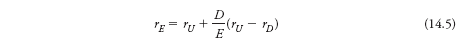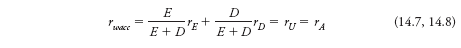# Data Case You work in the corporate finance division of The Home Depot and your boss has asked you to review the firm’s capital structure. Specifically, your boss is considering changing the firm’s debt level. Your boss remembers something from his MBA program about capital structure being irrelevant, but isn’t quite sure what that means. You know that capital structure is irrelevant under the conditions of perfect markets and will demonstrate this point for your boss by showing that the weighted average cost of capital remains constant under various levels of debt. So, for now, suppose that capital markets are perfect as you prepare responses for your boss. You would like to analyze relatively modest changes to Home Depot’s capital structure. You would like to consider two scenarios: the firm issues \$1 billion in new debt to repurchase stock, and the firm issues \$1 billion in new stock to repurchase debt. Use Excel to answer the following questions using Eq. 14.5 and Eq. 14.7 from this chapter (see equations below), and assuming a cost of unlevered equity (rU) of 12 percent. Obtain the financial information you need for Home Depot. Go to Nasdaq.com (http://www.nasdaq.com), click on “Summary Quotes” on the left-hand side, and enter Home Depot’s stock symbol (HD). Click on Go. From the Summary Quotes page, get the current stock price and number of shares outstanding. Click on “Company Financials” and the annual income statement should appear. Put the cursor in the middle of the statement, right-click your mouse, and select “Export to Microsoft Excel.” (You will not need the income statement until Chapter 15, but collect all of the background data in one step.) Go back to the Nasdaq Web page and select the balance sheet. Export that to Excel as well and then cut and paste the balance sheet to the same worksheet as the income statement. To get the cost of debt for Home Depot, go to NASD BondInfo (http://www.nasdbondinfo.com). Search by symbol and enter Home Depot’s symbol. The next page will contain information for all of Home Depot’s outstanding and recently matured bonds. Select the latest yield on an outstanding bond with the shortest remaining maturity (the maturity date is on the line describing each issue; sometimes the list also contains recently retired bonds, so make sure not to use one of those). Compute the market D/E ratio for Home Depot. Approximate the market value of debt by the book value of net debt; include both Long-Term Debt and Short-Term Debt/Current Portion of Long-Term Debt from the balance sheet and subtract any cash holdings. Use the stock price and number of shares outstanding to calculate the market value of equity. Compute the cost of levered equity (rE) for Home Depot using their current market debt-to-equity ratio and Eq. 14.5 from the chapter. Compute the current weighted average cost of capital (WACC) for Home Depot using Eq. 14.7 and the existing yield on the outstanding bonds as rD given their current debt-to-equity ratio. Repeat steps 3 and 4 for the two scenarios you would like to analyze, issuing \$1 billion in debt to repurchase stock, and issuing \$1 billion in stock to repurchase debt. (Although you realize that the cost of debt capital (rD) may change with changes in leverage, for these modestly small changes you decide to assume that rD remains constant. We will explore the relation between changing leverage and changing rD more fully in Chapter 16.) What is the market D/E ratio in each of these cases? Prepare a written explanation for your boss explaining the relationship between capital structure and the cost of capital in this exercise. What implicit assumptions in this exercise generate the results found in question 5? How might your results differ in the “real world”? Equations: 14.5 – According to MM Proposition II, the cost of capital for levered equity is: Equation 14.5 14.7 – Debt is less risky than equity, so it has a lower cost of capital. Leverage increases the risk of equity, however, raising the equity cost of capital. The benefit of debt’s lower cost of capital is offset by the higher equity cost of capital, leaving a firm’s weighted average cost of capital (WACC) unchanged with perfect capital markets: Equation 14.7 and 14.8

Data Case

You work in the corporate finance division of The Home Depot and your boss has asked you to review the firm’s capital structure. Specifically, your boss is considering changing the firm’s debt level. Your boss remembers something from his MBA program about capital structure being irrelevant, but isn’t quite sure what that means. You know that capital structure is irrelevant under the conditions of perfect markets and will demonstrate this point for your boss by showing that the weighted average cost of capital remains constant under various levels of debt. So, for now, suppose that capital markets are perfect as you prepare responses for your boss.

You would like to analyze relatively modest changes to Home Depot’s capital structure. You would like to consider two scenarios: the firm issues \$1 billion in new debt to repurchase stock, and the firm issues \$1 billion in new stock to repurchase debt. Use Excel to answer the following questions using Eq. 14.5 and Eq. 14.7 from this chapter (see equations below), and assuming a cost of unlevered equity (rU) of 12 percent.

1. Obtain the financial information you need for Home Depot.
1. Go to Nasdaq.com (http://www.nasdaq.com), click on “Summary Quotes” on the left-hand side, and enter Home Depot’s stock symbol (HD). Click on Go. From the Summary Quotes page, get the current stock price and number of shares outstanding.
2. Click on “Company Financials” and the annual income statement should appear. Put the cursor in the middle of the statement, right-click your mouse, and select “Export to Microsoft Excel.” (You will not need the income statement until Chapter 15, but collect all of the background data in one step.) Go back to the Nasdaq Web page and select the balance sheet. Export that to Excel as well and then cut and paste the balance sheet to the same worksheet as the income statement.
3. To get the cost of debt for Home Depot, go to NASD BondInfo (http://www.nasdbondinfo.com). Search by symbol and enter Home Depot’s symbol. The next page will contain information for all of Home Depot’s outstanding and recently matured bonds. Select the latest yield on an outstanding bond with the shortest remaining maturity (the maturity date is on the line describing each issue; sometimes the list also contains recently retired bonds, so make sure not to use one of those).
2. Compute the market D/E ratio for Home Depot. Approximate the market value of debt by the book value of net debt; include both Long-Term Debt and Short-Term Debt/Current Portion of Long-Term Debt from the balance sheet and subtract any cash holdings. Use the stock price and number of shares outstanding to calculate the market value of equity.
3. Compute the cost of levered equity (rE) for Home Depot using their current market debt-to-equity ratio and Eq. 14.5 from the chapter.
4. Compute the current weighted average cost of capital (WACC) for Home Depot using Eq. 14.7 and the existing yield on the outstanding bonds as rD given their current debt-to-equity ratio.
5. Repeat steps 3 and 4 for the two scenarios you would like to analyze, issuing \$1 billion in debt to repurchase stock, and issuing \$1 billion in stock to repurchase debt. (Although you realize that the cost of debt capital (rD) may change with changes in leverage, for these modestly small changes you decide to assume that rD remains constant. We will explore the relation between changing leverage and changing rD more fully in Chapter 16.) What is the market D/E ratio in each of these cases?
6. Prepare a written explanation for your boss explaining the relationship between capital structure and the cost of capital in this exercise.
7. What implicit assumptions in this exercise generate the results found in question 5? How might your results differ in the “real world”?

Equations:

14.5 – According to MM Proposition II, the cost of capital for levered equity is:14.7 – Debt is less risky than equity, so it has a lower cost of capital. Leverage increases the risk of equity, however, raising the equity cost of capital. The benefit of debt’s lower cost of capital is offset by the higher equity cost of capital, leaving a firm’s weighted average cost of capital (WACC) unchanged with perfect capital markets: